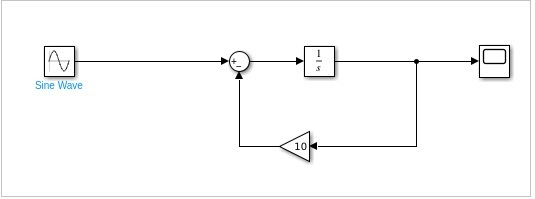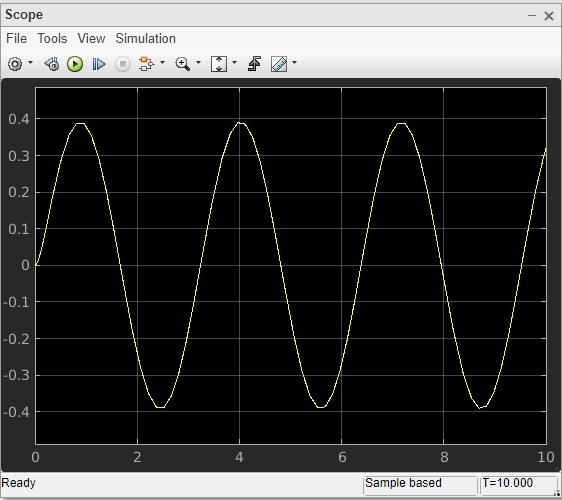# First Order Differential Equation

#### Data Preprocessing for Machine Learning using MATLAB

30 Lectures 4 hours

#### Image Processing Toolbox in MATLAB

17 Lectures 3 hours

#### Matlab - The Complete Course

Best Seller

37 Lectures 5 hours

Here, we will learn how to solve the first order differential equation in Simulink.

The first order differential equation that we are trying to solve using Simulink is as follows −

```dy/dt = 4sin2t - 10y
```

The equation can be solved by integrating dy/dt to the following −

y(t)=∫(4sin2t - 10y(t))dt

Following are the steps to build a model for above equation.

• Pick up sine wave from sources library and change the amplitude to 4 and frequency to 2. This will give us 4sin2t.

• The integrator block will be used to show dy/dt that will give output y(t).

• The gain block will represent 10y.

• The input of step 1 and 3 will be given to step2.

• We need scope block to see the output y(t). The step 4 will be connected to the scope block.

Let us see the above steps in model as shown below −Run the block to see the following output −